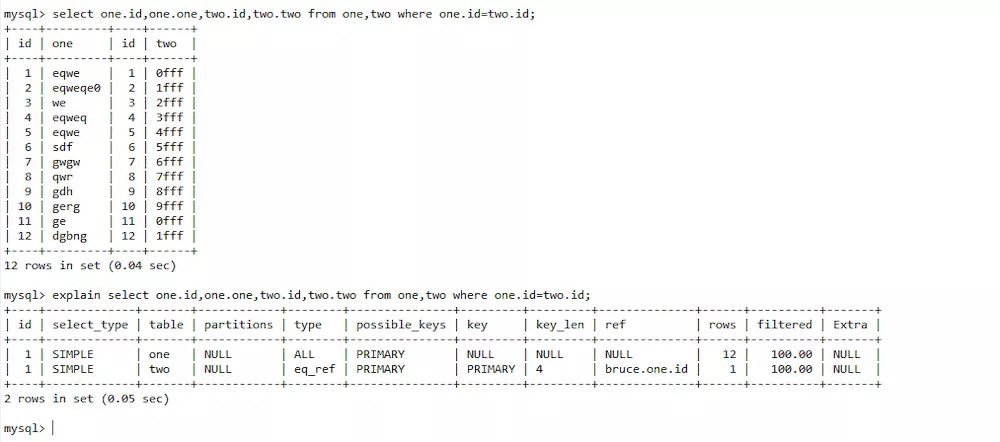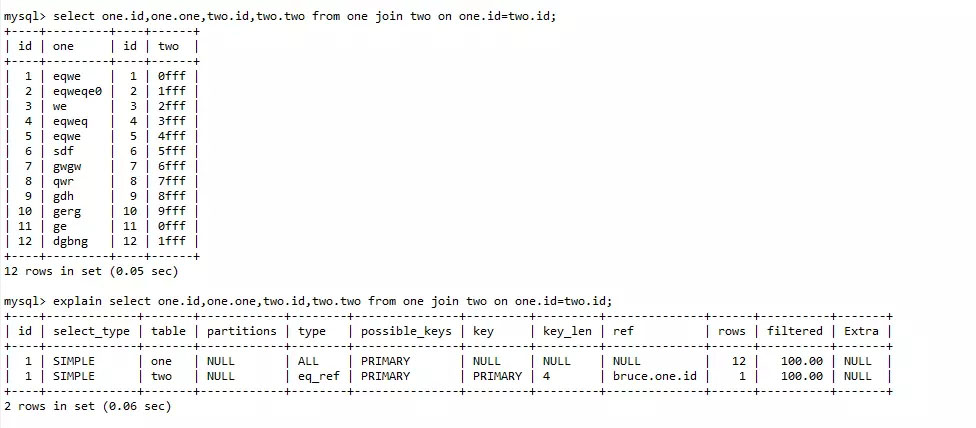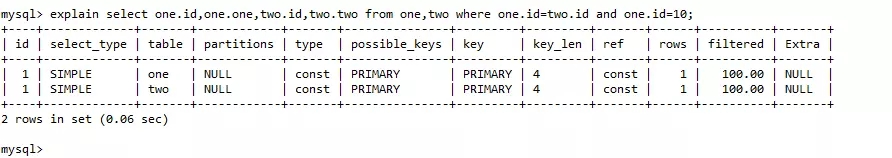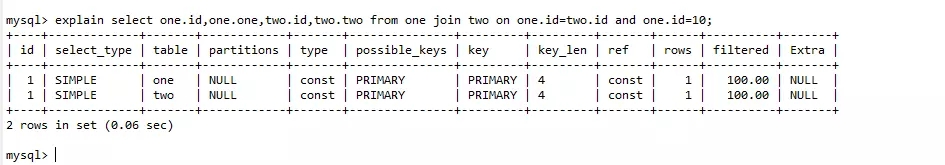## mysql使用from与join两表查询的区别总结

1.先在本地的mysql上先建两个表one和two

one表

?

 1 2 3 4 5 `CREATE` `TABLE` ``one` (` ` ```id` ``int``(0) ``NOT` `NULL` `AUTO_INCREMENT,` ` ```one` ``varchar``(100) ``NOT` `NULL``,` ` ``PRIMARY` `KEY` `(`id`)` `) ENGINE = InnoDB ``CHARACTER` `SET` `= utf8;`

two表

?

 1 2 3 4 5 `CREATE` `TABLE` ``two` (` ` ```id` ``int``(0) ``NOT` `NULL` `AUTO_INCREMENT,` ` ```two` ``varchar``(100) ``NOT` `NULL``,` ` ``PRIMARY` `KEY` `(`id`)` `) ENGINE = InnoDB ``CHARACTER` `SET` `= utf8;`

?

 1 `select` `one.id,one.one,two.id,two.two ``from` `one,two ``where` `one.id=two.id;`?

 1 `select` `one.id,one.one,two.id,two.two ``from` `one ``join` `two ``on` `one.id=two.id;`?

 1 2 3 4 5 6 7 8 9 10 11 12 13 14 15 16 17 18 19 20 21 22 `import pymysql`   `db = pymysql.``connect``(``"127.0.0.1"``, ``'root'``, ``"123456"``, ``"bruce"``)` `cursor` `= db.``cursor``()`   `sql = ``"INSERT INTO one (one) values (%s)"` `for` `i ``in` `range(1000000):` ` ``cursor``.executemany(sql, [``'one'` `+ str(i)])` ` ``if i % 10000 == 0:` ` ``db.``commit``()` ` ``print(str(i) + ``'次 commit'``)` `db.``commit``()`   `print(``'insert one ok'``)` `sql2 = ``"INSERT INTO two (two) values (%s)"` `for` `i ``in` `range(100000):` ` ``cursor``.executemany(sql2, [``'two'` `+ str(i)])` ` ``if i % 10000 == 0:` ` ``db.``commit``()` ` ``print(str(i) + ``'次 commit'``)` `db.``commit``()` `print(``'insert two ok'``)`

?

 1 `select` `one.id,one.one,two.id,two.two ``from` `one,two ``where` `one.id=two.id;`?

 1 `select` `one.id,one.one,two.id,two.two ``from` `one ``join` `two ``on` `one.id=two.id;`